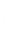# Solving Systems of Equations: EliminationIn this lesson we will discuss how to solve systems of equations using the elimination method. The elimination method “eliminates” one of the variables given in order to find the remaining. Below is a easy step process of how to solve problems using the elimination method.

### Example 1:

2x – 4y = 4

2x – 6y = 10

First decide which variable you would like to eliminate first. In this case we are going to eliminate x first. The coefficient in front of the x variable is the same which makes it easier to eliminate.

2x – 4y = 4

2x – 6y = 10In order to eliminate the x variable we must multiply one of the equations (entirely) by -1. It is completely up to you on which equation you choose to multiply by -1. In this case we decided to go with the first equation.

After multiplying the first equation by -1 it should be rewritten like this:

Now that we have canceled the x variables from both equations we can combine like-terms and solve for y.

Now we have calculated what y is equal to. We can substitute the value of y back into one of the original equations (the decision is up to you) to calculate the value of x.

The final answer is (-4, -3)

### Example 2:

-3x + 2y = 6

3x + 4y = 9

In this example, we can eliminate the x variable since that is the easiest approach.

-3x + 2y = 6

3x + 4y = 9

After eliminating the x variable the equations should state:

2y = 6

4y = 9

Now combine like-terms and solve for y.

We must now plug the value for y back into one of the original equations to find x. In this case we will pick the second equation.

## Follow us on our Instagram page @scholastophere and our YouTube channel for more content and educational videos.

#### Ready to learn with Scholastophere?